# 月之暗面

The DARKSIDE of the Moon

0%

## 断断续续的无效工作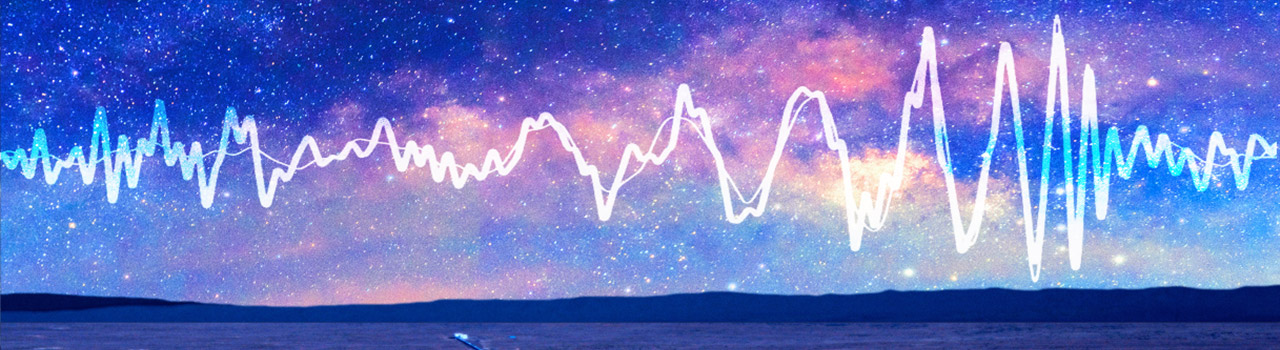# EmoeMetrology测试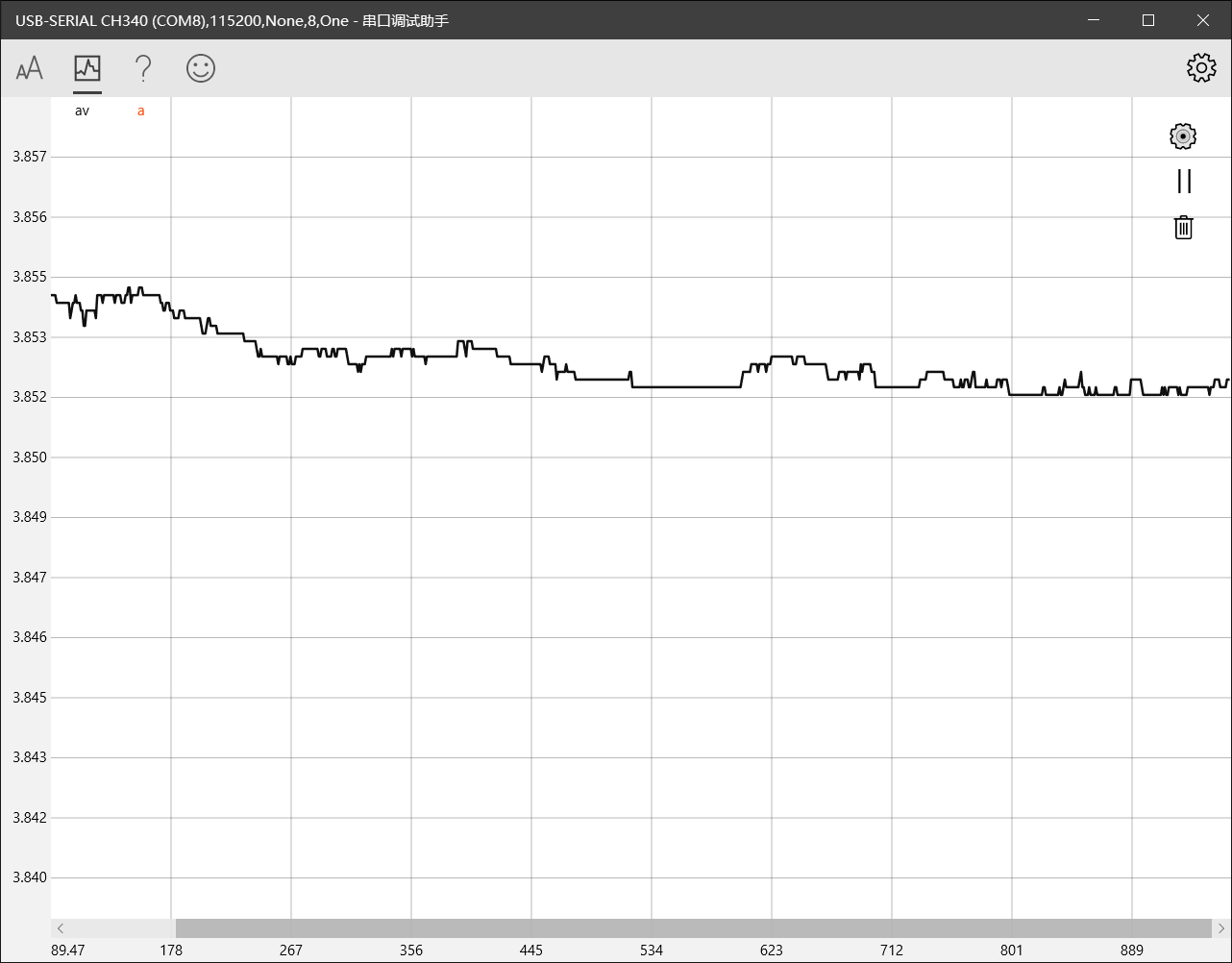2月14号坐飞机提前润回了学校。在家中呆着我只感觉压抑，虽然与家人相处和睦，但他们一贯的性格，或者说是我的性格，让我没有与他们交流的欲望...每次交流都像是在承受某种煎熬。顺便，我现在跟不太熟的人尬聊也是这种感觉。嗯，为什么是情人节那天回呢，因为那天机票最便宜，只要400多。

# 基础模拟与数字调制技术

sorry,我仔细看了那篇教程后发现其并不是特别适合写这篇文。那么我就从Wiki和各种参考书和教材上搜集资料了。

# Working List

• AM
• FM
• PM
• FSK
• PSK
• 特殊信号
• 高阶调制 鸽鸽还在咕😭
• 其他 s # 为什么要调制？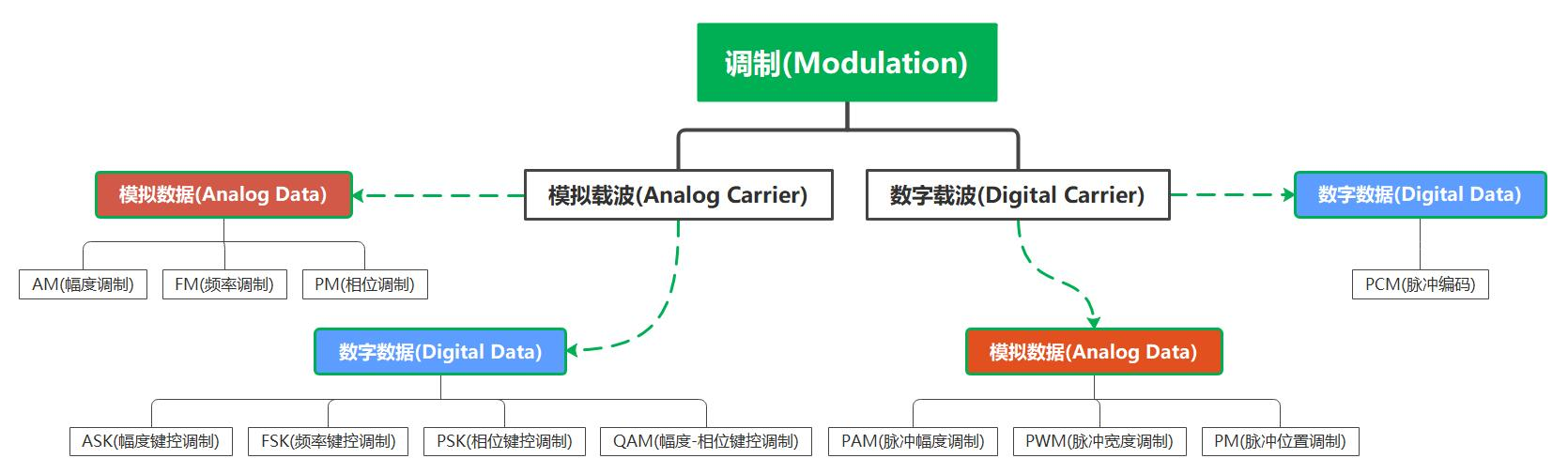Written by markdown & LATEX, Coding by python.numpy & matplotlib

## LTI系统对复指数信号的响应

$连续时间: e^{st} \rightarrow H(s)e^{st} \cdots (3.1)\\ 离散时间: z^n \rightarrow H(z)z^n \cdots (3.2)$

### 证明复指数是LTI系统的特征函数

$y(t) = \int_{-\infty}^{+\infty} h(T)x(t-T)dT = \int_{-\infty}^{+\infty} h(T)e^{s(t-T)}dT \cdots(3.3)$

$$e^{s(t-T)}$$可写成$$e^{st}e^{-sT}$$，而$$e^{st}$$可以从积分号内移出来，这样（3.3）式变成:

$y(t) = e^{st} \int_{-\infty}^{+\infty} h(T)e^{-sT}dT \cdots (3.4)$

# 深入理解DDS（数字频率合成）

🐟最近也在研究DDS（毕设）,所以闲来无事翻译翻译。。

## Section 2.理解DDS的采样输出特性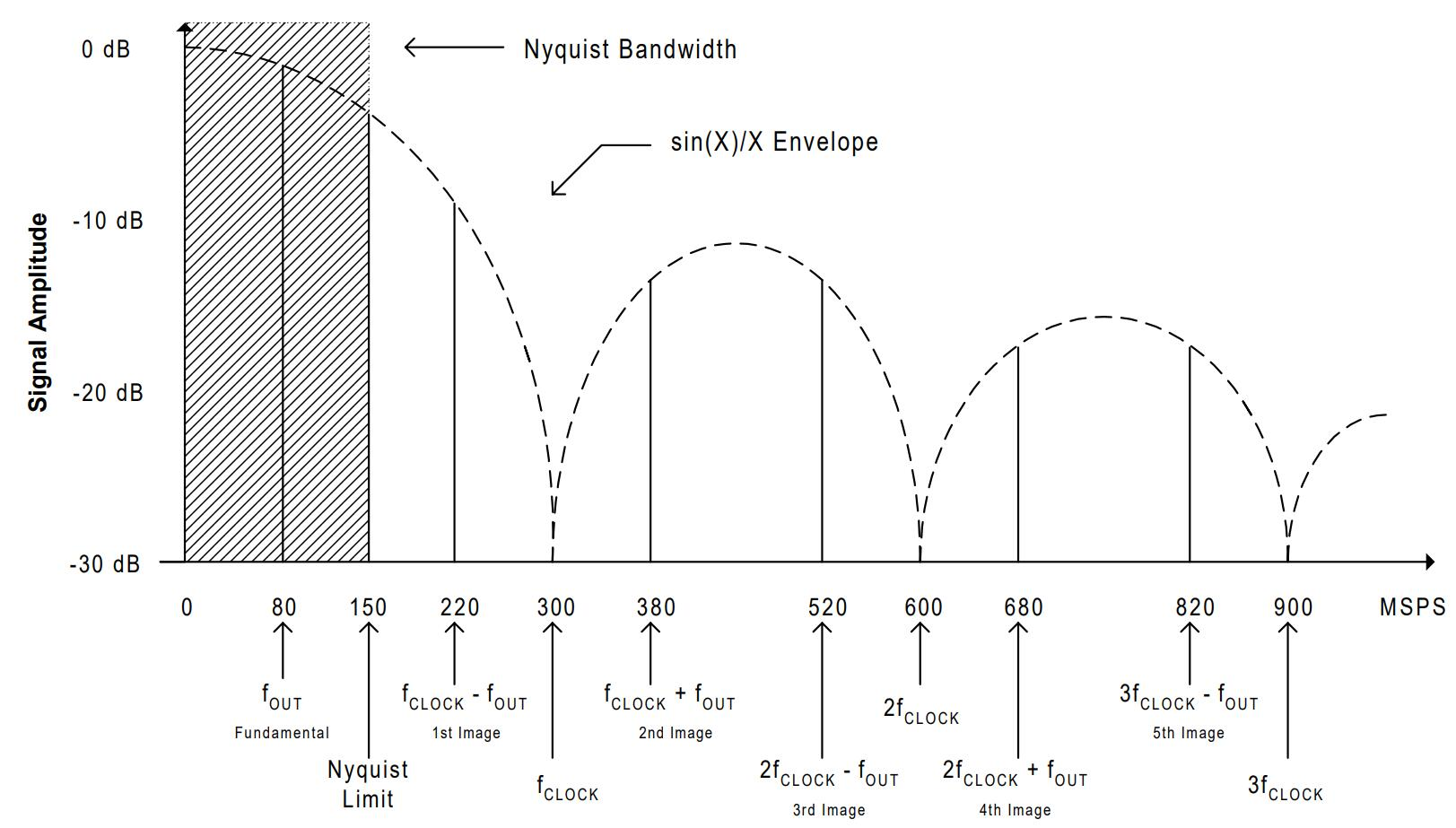"采样"输出的频谱成分

=================## 纹波（Ripple）与噪声（Noise）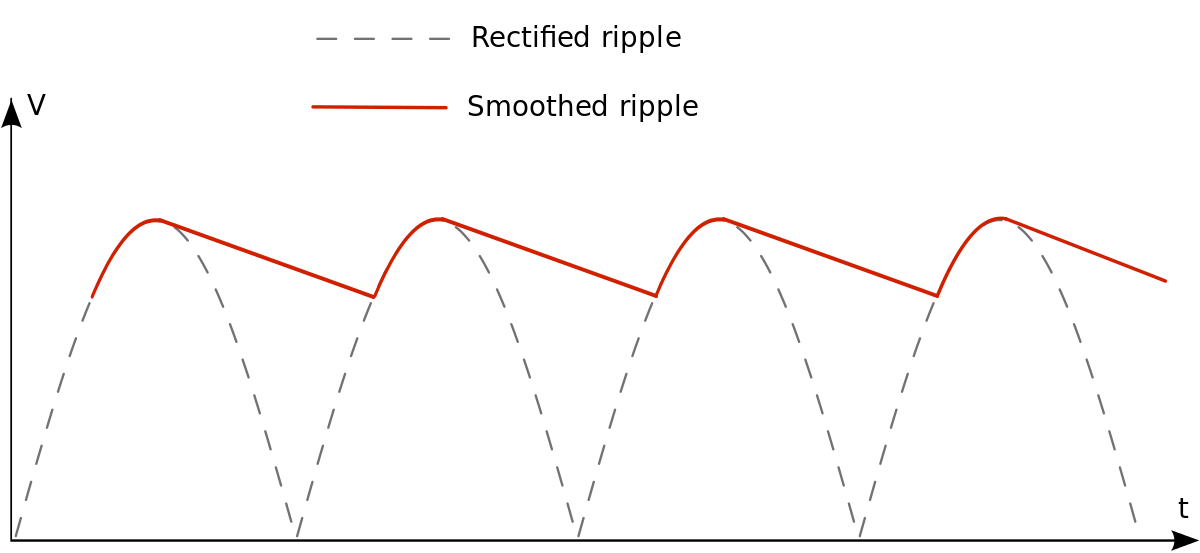• 大气层
• 外太空
• 人为噪声